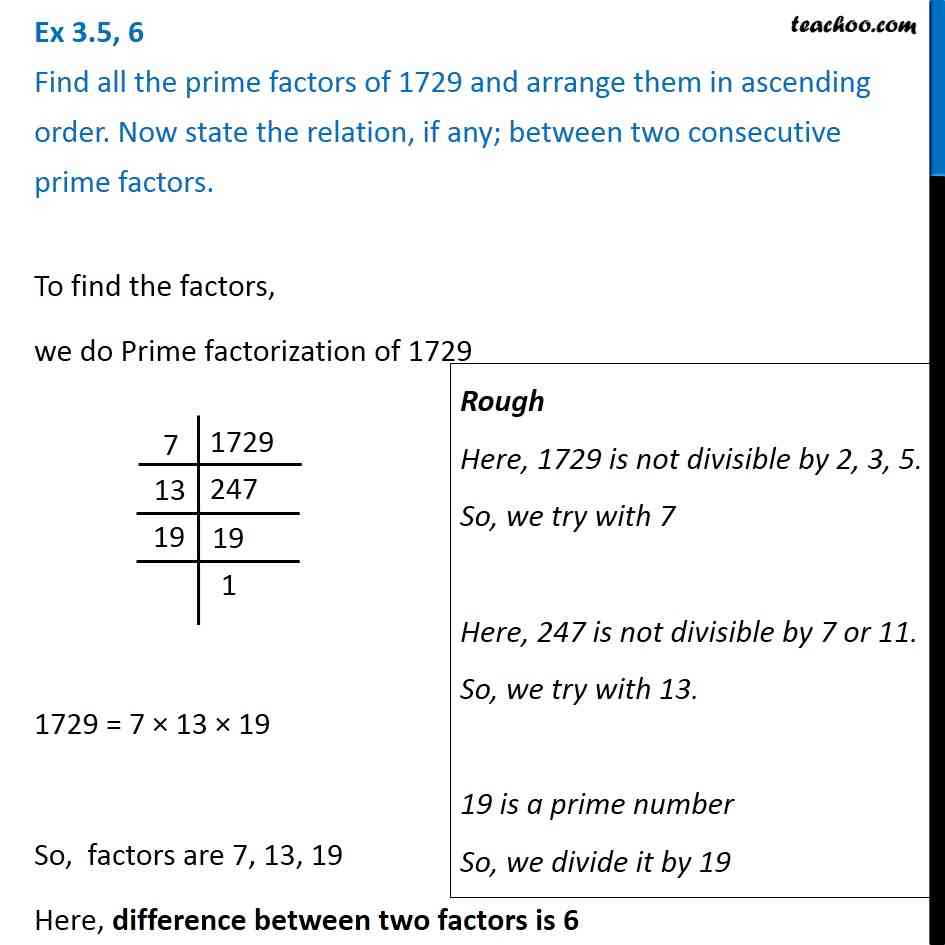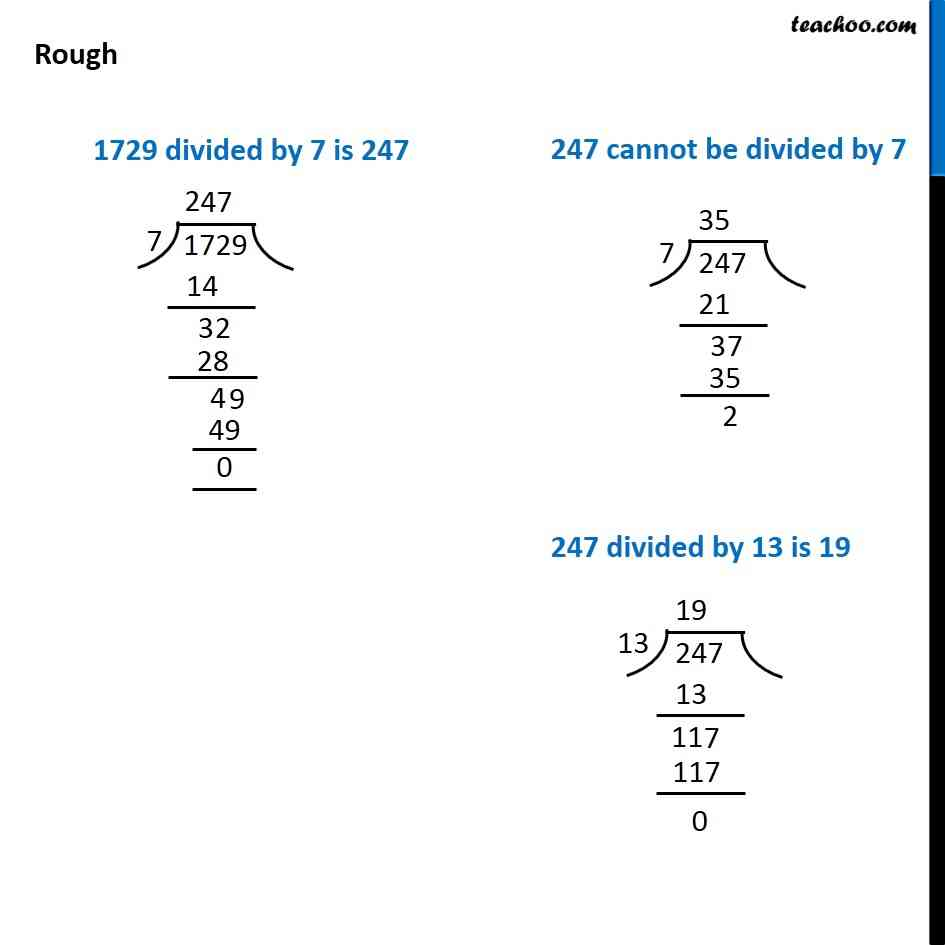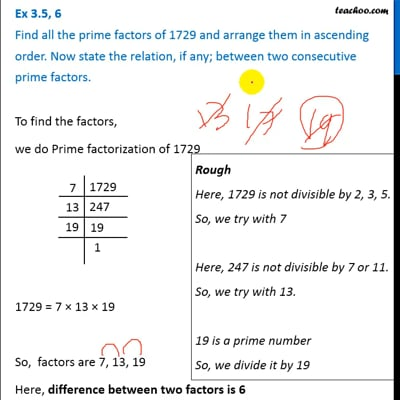Ex 3.5

Chapter 3 Class 6 Playing with Numbers
Serial order wiseThis video is only available for Teachoo black users

Introducing your new favourite teacher - Teachoo Black, at only ₹83 per month

### Transcript

Ex 3.5, 6 Find all the prime factors of 1729 and arrange them in ascending order. Now state the relation, if any; between two consecutive prime factors.To find the factors, we do Prime factorization of 1729 1729 = 7 × 13 × 19 So, factors are 7, 13, 19 Here, difference between two factors is 6 Rough Here, 1729 is not divisible by 2, 3, 5. So, we try with 7 Here, 247 is not divisible by 7 or 11. So, we try with 13. 19 is a prime number So, we divide it by 19 1729 divided by 7 is 247 247 cannot be divided by 7 247 divided by 13 is 19